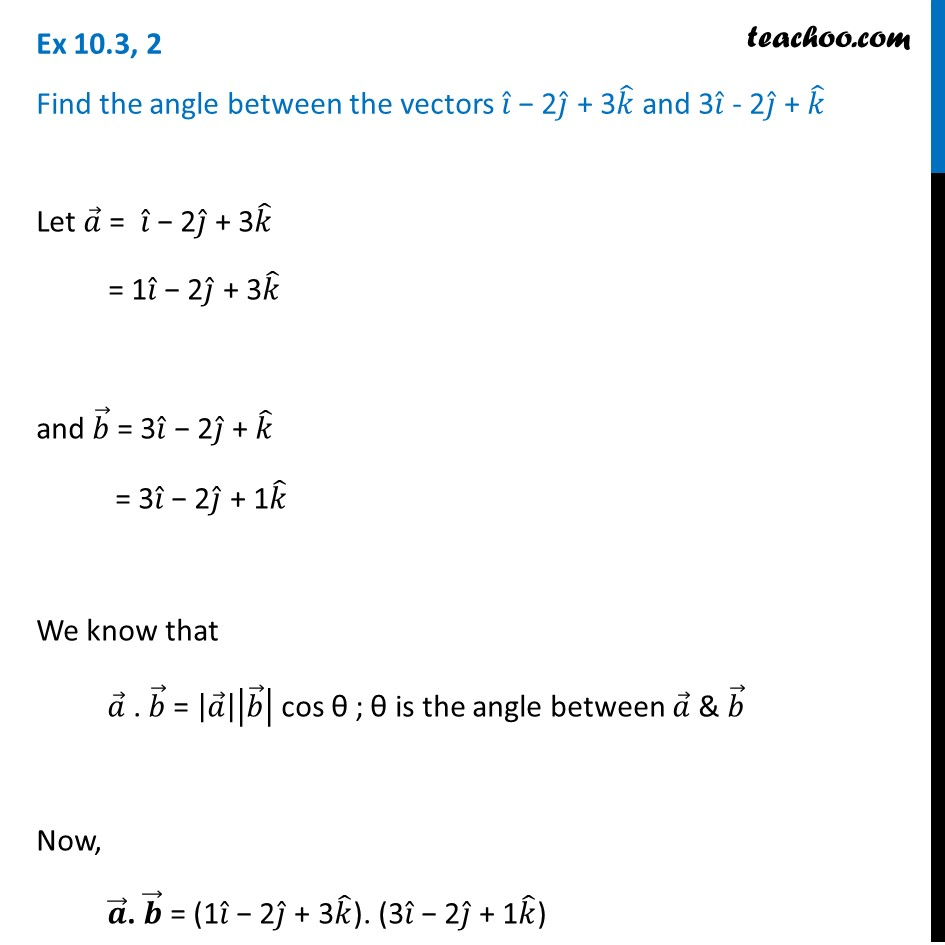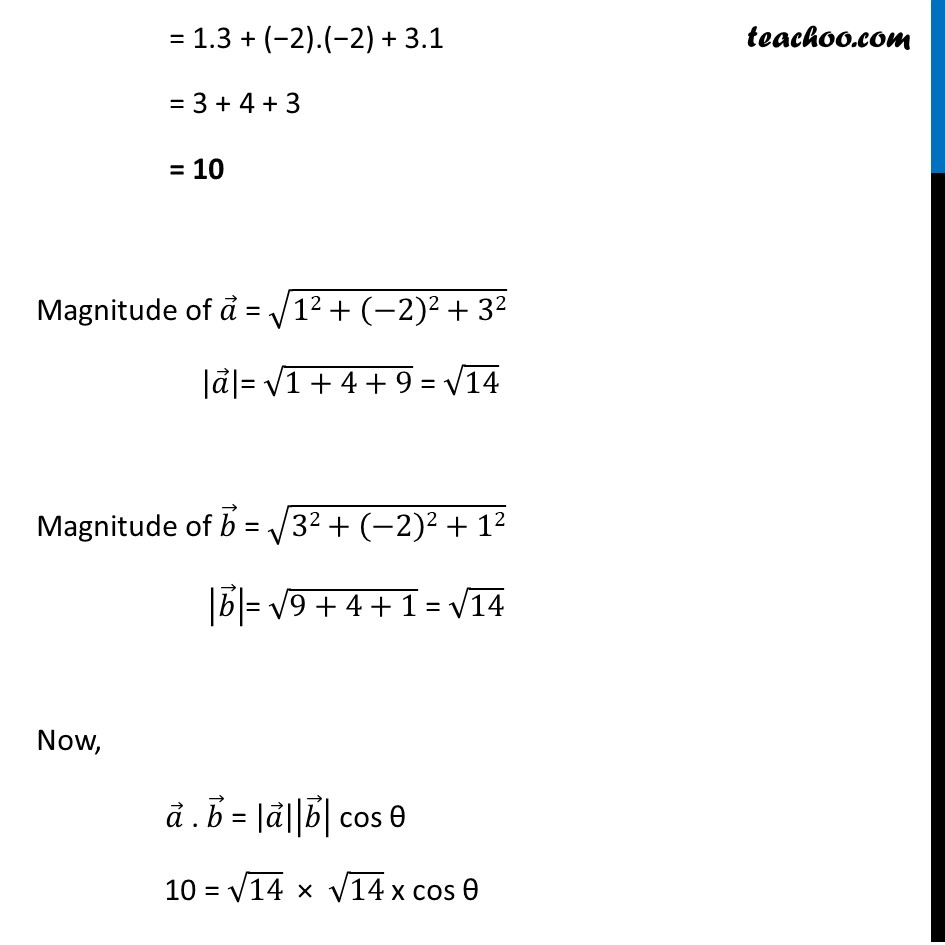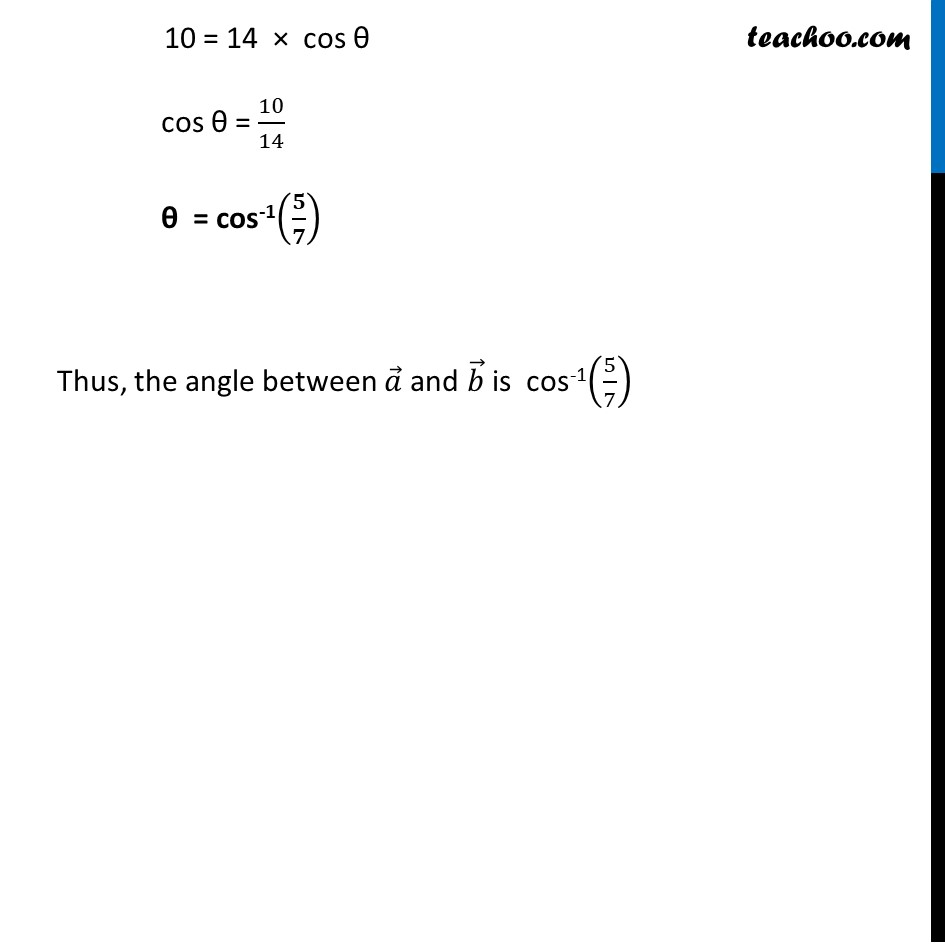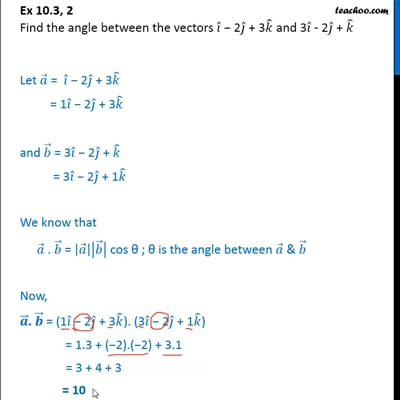Scalar product - Defination

Chapter 10 Class 12 Vector Algebra
Concept wiseThis video is only available for Teachoo black users

Introducing your new favourite teacher - Teachoo Black, at only ₹83 per month

### Transcript

Ex 10.3, 2 Find the angle between the vectors 𝑖 ̂ − 2𝑗 ̂ + 3𝑘 ̂ and 3𝑖 ̂ - 2𝑗 ̂ + 𝑘 ̂Let 𝑎 ⃗ = 𝑖 ̂ − 2𝑗 ̂ + 3𝑘 ̂ = 1𝑖 ̂ − 2𝑗 ̂ + 3𝑘 ̂ and 𝑏 ⃗ = 3𝑖 ̂ − 2𝑗 ̂ + 𝑘 ̂ = 3𝑖 ̂ − 2𝑗 ̂ + 1𝑘 ̂ We know that 𝑎 ⃗ . 𝑏 ⃗ = |𝑎 ⃗ ||𝑏 ⃗ | cos θ ; θ is the angle between 𝑎 ⃗ & 𝑏 ⃗ Now, 𝒂 ⃗. 𝒃 ⃗ = (1𝑖 ̂ − 2𝑗 ̂ + 3𝑘 ̂). (3𝑖 ̂ − 2𝑗 ̂ + 1𝑘 ̂) = 1.3 + (−2).(−2) + 3.1 = 3 + 4 + 3 = 10 Magnitude of 𝑎 ⃗ = √(12+(−2)2+32) |𝑎 ⃗ |= √(1+4+9) = √14 Magnitude of 𝑏 ⃗ = √(32+(−2)2+12) |𝑏 ⃗ |= √(9+4+1) = √14 Now, 𝑎 ⃗ . 𝑏 ⃗ = |𝑎 ⃗ ||𝑏 ⃗ | cos θ 10 = √14 × √14 x cos θ 10 = 14 × cos θ cos θ = 10/14 θ = cos-1(𝟓/𝟕) Thus, the angle between 𝑎 ⃗ and 𝑏 ⃗ is cos-1(5/7)